# How to Design and Build a Simple Monostable Multivibrator Circuit using Op-amp

Published  December 14, 2020   0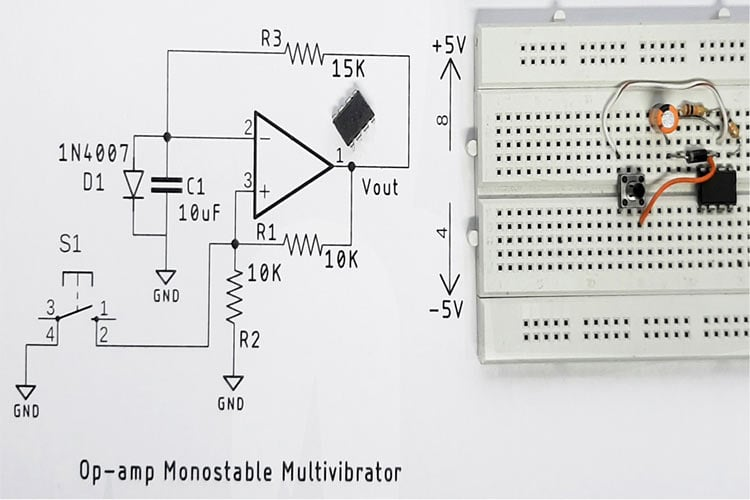An operational amplifier or Op-amp is one of the most commonly used components in any electronic design. It’s a very versatile device that can be used for a wide variety of applications. In our previous projects, we put the op-amps capabilities to the test by making a ton of projects with it, you can check those out if you want to explore more on the topic. We have also covered basic op-amp circuits like the Summing Amplifier, Differential Amplifier, Instrumentation Amplifier, Voltage Follower, Op-Amp Integrator, etc. In this tutorial, we will be making an Op-amp based monostable multivibrator circuit along with all its calculations, and testing. So let’s get right into it.

### What is Monostable Multivibrator -The Basics

A monostable multivibrator is also called a single short multivibrator, it has only one stable state and the other state is a quasi-stable state. In the previous project, we took the opportunity to make a NE555 based monostable multivibrator circuit. You can check that out if you are looking for something like that. By stable state in a multivibrator, we mean that at that moment, the output is high or low. Now, when a triggering pulse is applied, the multivibrator changes its output from a stable state to a quasi-stable state. And after a certain time 'T', which is determined by the circuit components, the multivibrator returns to its original stable state automatically. In other words, no external triggering signal is required to induce this reverse transition. The circuit remains in this state until another triggering pulse is applied. These multivibrators are also called one-shot, single cycle, single-step, or unified multivibrator. Time ‘T’ after which the circuit returns to its original state is called gate-width and hence the multivibrator is also known as the gating circuit or delay circuit. This circuit is used to produce the pulses of variable width at the required moment. The image below will give you a better idea about the topic.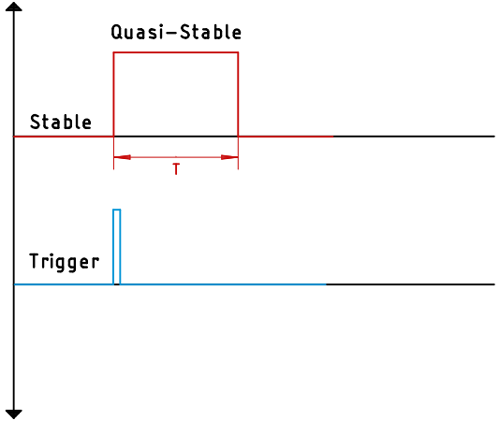Now that we have a clear idea of what monostable multivibrator is, let’s see how we can design a monostable multivibrator circuit using Op-amp.

### Monostable Multivibrator with Op-amp - Working

In order to fully understand the working principle of the monostable multivibrator, you first need to understand the working principle of the Schmitt trigger circuit, and in a previous post on the Astable multivibrator circuit, we have discussed the topic of Schmitt trigger in extreme details. You should check that out if you do not know about a Schmitt trigger circuit.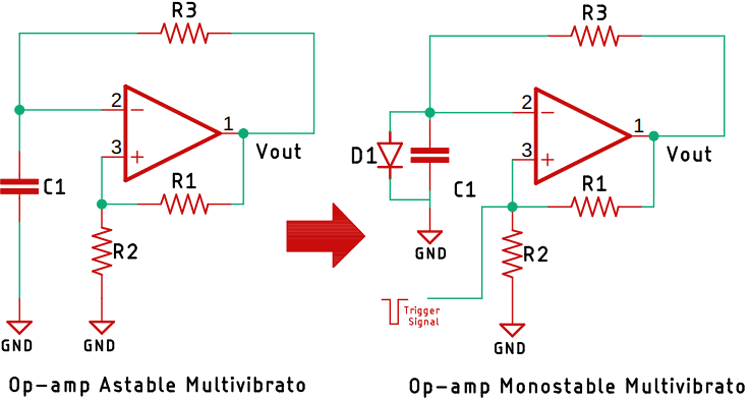If you have read the article about an astable multivibrator circuit, you already know about it. As you can see by slightly modifying an astable multivibrator circuit, or by just putting a diode in place, we have converted the astable circuit into a monostable circuit. And as you can see in the above image, a diode is connected in parallel to the capacitor and the triggering signal is applied at that node where R1 and R2 get connected. So, let’s understand the working principle of this circuit.

Let's consider the output is at positive saturation voltage or the output of the op-amp is positive, then the voltage at node A will be (R2/(R1+R2)) * Vsat, and whenever the output is at positive saturation voltage, the diode D1 will become forward biased, and the voltage across the capacitor C1 will be the forward voltage drop across the diode. So, at the inverting node, the voltage will be equal to the forward voltage drop across the diode. For this scenario, as the output voltage is greater than the inverting node, the output of the op-amp will be at the positive saturation voltage.

Now we will apply a trigger pulse at node A, and whenever this negative trigger signal has applied, the output at the non-inverting node will be less than the voltage at the inverting node. And the output of the op-amp will switch from the positive saturation voltage to the negative saturation voltage. And the circuit will go into the quasi-stable state.

Now the voltage at the non-inverting node will be equal to -XVsat. As the output voltage is equal to the negative saturation voltage, the diode will become reversed biased and the capacitor will start charging towards the negative saturation voltage. Now whenever the voltage at the inverting node goes below the voltage -XVsat, then once again the output of the op-amp will become positive saturation voltage because, at that time, the non-inverting node will be slightly less than the inverting node. So, the output will switch from negative saturation voltage to positive saturation voltage. And for this time T, only the output will stay on the quasi-stable as soon as the output reaches the positive saturation voltage, the diode will become forward biased and this cycle will continue. So this is the basic working principle of the monostable multivibrator. Now as we have understood the working principle, we can now move onto calculating the time duration of the pulse.

### Monostable Multivibrator using Op-amp Applications

The monostable multivibrator is a very important circuit and is used practically in many designs even today. The most common application of a monostable multivibrator is in delay and timing circuits. Apart from that, it can also be found to be used in trigger circuits, pulse correctors, and even in memory circuits.

### Op-amp Monostable Multivibrator using Op-Amp derivation

In this circuit also, the period ‘T’ is determined by the values of the resistor R3, the capacitor C1, and the values of the feedback resistors, and for the given circuit, the time-period can be calculated by-

`T = RC * logn (1 + (R2 / R1))`

With the formula, sorted outlets design a monostable multivibrator that has a time-period of 100ms. For simplicity, we are going to be using two 10K resistors for R1 and R2. By doing so, the expression becomes-

```T = RC * logn (1 + 1)
T = RC * logn (2)```

If we were to put these values on the calculator and calculate the values, it becomes 0.693RC and for the capacitor, we are going to use a 10uF capacitor.

```T = 0.693 RC
C = 10 uF
R = 15 K```

Now, as we have completed the calculations, we can move on to the building and testing the circuit.

### Monostable Multivibrator using op-amp Experiment

Now that we have understood the basics of a Monostable Multivibrator and how it can be designed using an op-amp, let's actually build a small circuit to experiment with it.

### Components Required

As this is a very simple monostable multivibrator circuit, the component requirements for this project are very simple, and you can get those from your local hobby store. The list of components is given below.

• LM358 Op-amp IC - 1
• 10K Resistors - 3
• 4.7K Resistor - 1
• 0.1uF Capacitor - 2
• 1N4007 Diode - 4
• 1000uF, 25V Capacitors - 2
• 4.5V - 0 - 4.5V Transformer - 1
• AC Cable - 1
• Connecting Wires

### Schematic for Op-amp Monostable Multivibrator

The complete circuit diagram for the Op-amp based Monostable Multivibrator Circuit with values is given below.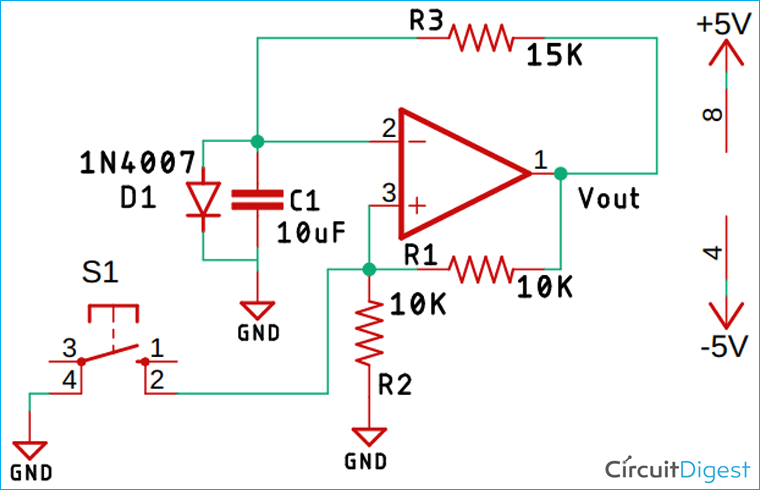As you can see, we have used the LM358 to build this circuit, but you can also build a monostable multivibrator using the IC741, both will have the same circuit and should work similarly.

### Testing of the Op-amp Monostable Multivibrator Circuit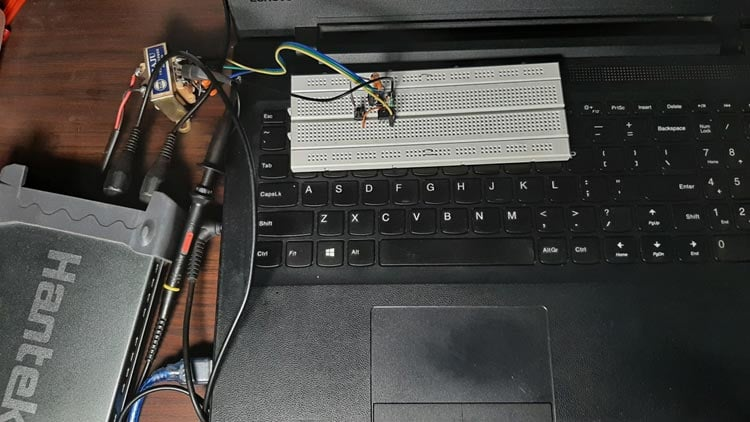The test setup for the Op-amp based monostable multivibrator circuit is shown above, as you can see, we have used a transformer with four diodes and two capacitors to produce a dual polarity supply, and we have used three 10K resistor, one 4.7K resistor, one 10uF capacitor, and a pushbutton to build the circuit, the LM358 Op-amp. A clear image of the circuit is shown below.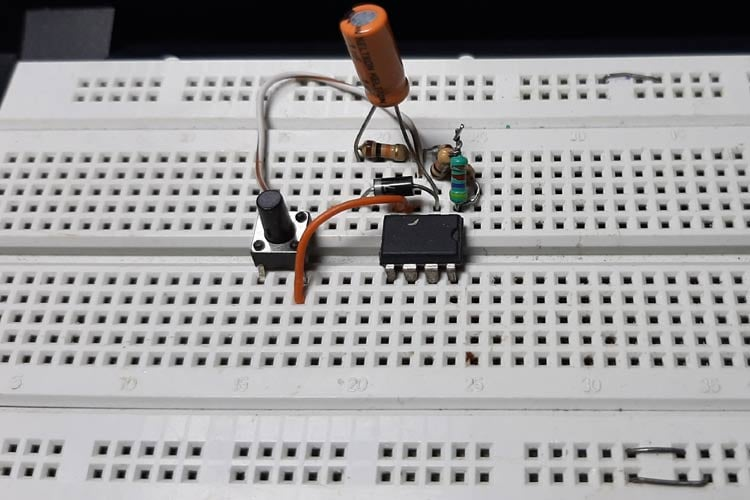After the circuit was complete, I pulled out my Hantek oscilloscope to measure the time of the output pulse, and as you can see from the image below, it was around 180ms because of the value of the resistor and capacitor selected.  That concludes the project, a snapshot of the output is shown below. You can check out the video at the bottom of the page for a clear explanation.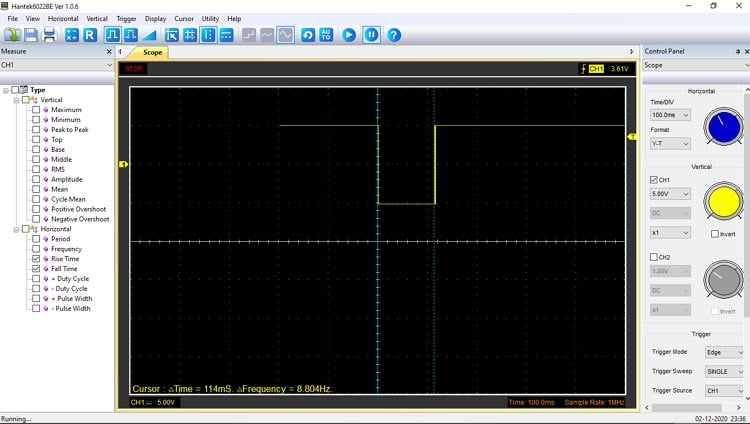I hope you liked the article and learned something new, if you have any questions regarding the article, you can ask in our forum.

Video